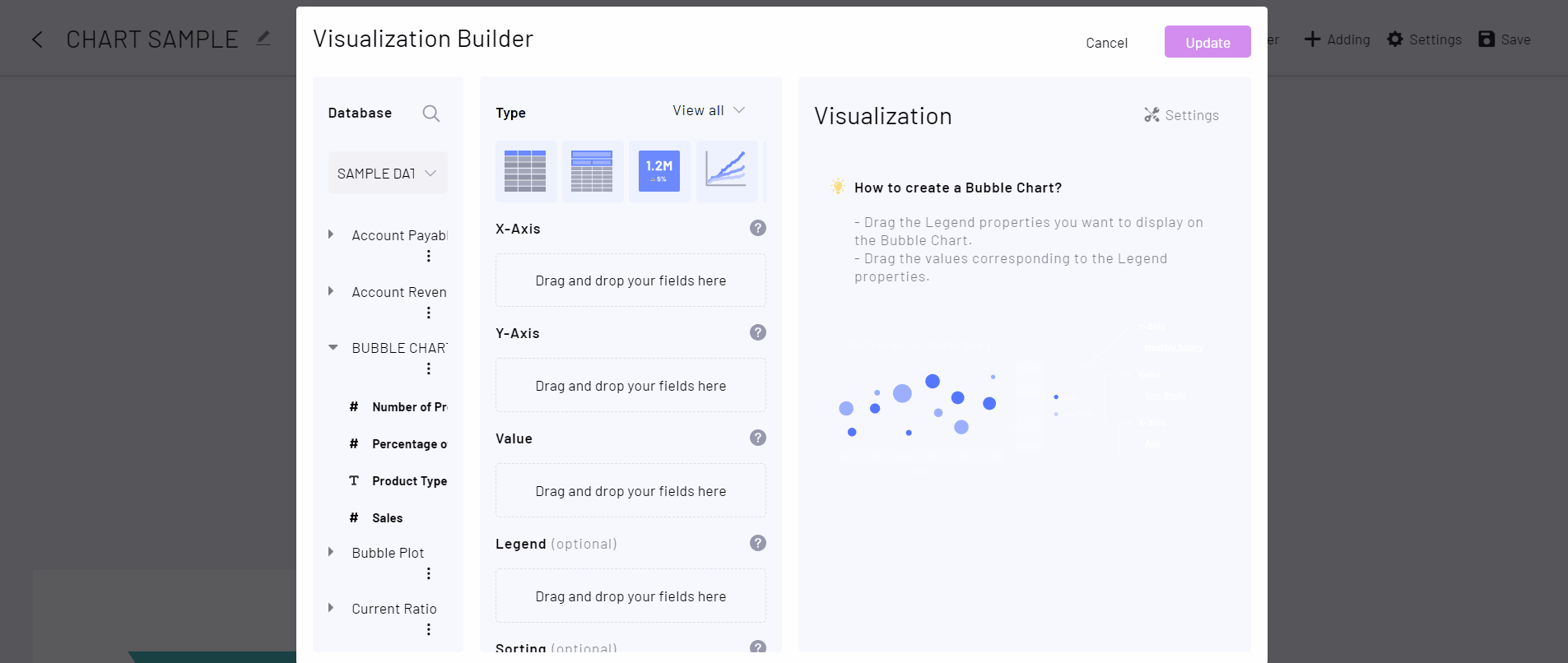top of page
Search
• Tracy Tran

# Bubble Plot in DataInsider

Updated: Aug 22, 2021

```Index
1. What is a Bubble Plot?
1. What is a Bubble Plot?
2. When to use a Bubble Plot?
2. When to use a Bubble Plot?
3. Step By Step Guidance with DataInsider3. Step By Step Guidance with DataInsider```

## 1. What is a Bubble Plot?

A Bubble Plot is a scatterplot where the value of an additional numeric variable is added. This value is represented by the size of the dots.## 2. When to use a Bubble Plot?

Consider using Bubble Plots when you have 3 numerical variables: one is represented by the X-axis, one by the Y-axis, and one by the dot size.

Bubble Plots is great at showing the relationship between 2 variables with additional information from another variable.

## 3. Step by step guidance with DataInsider

Desired chart: A Bubble Plot represents the relationship between Discount and Profit with the third dimension of Sales.

Step 1. Drag Number of Products to X-Axis

Step 2. Drag Sales to Y-Axis

Step 3. Drag Percent of Market Shareto Value

Step 4. Drag Product Type to LegendResult: